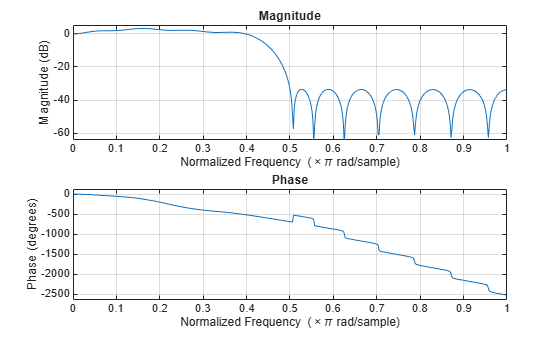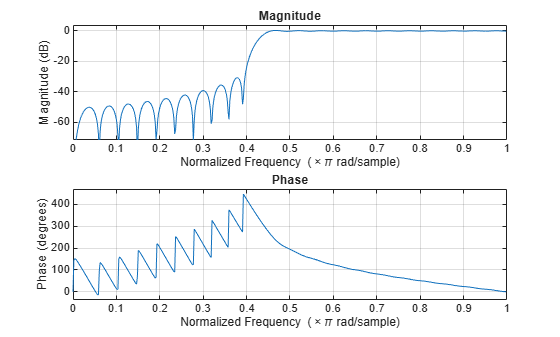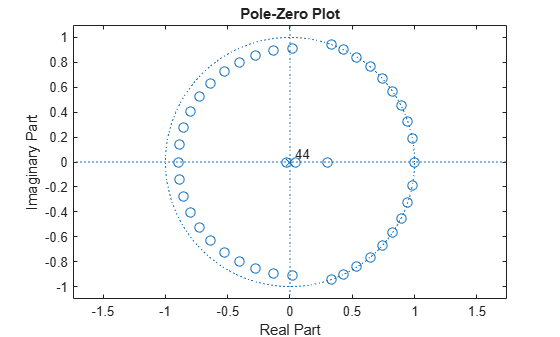# firlpnorm

Least P-norm optimal FIR filter

## Syntax

```b = firlpnorm(n,f,edges,a) b = firlpnorm(n,f,edges,a,w) b = firlpnorm(n,f,edges,a,w,p) b = firlpnorm(n,f,edges,a,w,p,dens) b = firlpnorm(n,f,edges,a,w,p,dens,initnum) b = firlpnorm(...,'minphase') [b,err] = firlpnorm(...) ```

## Description

`b = firlpnorm(n,f,edges,a)` returns a filter of numerator order `n` which represents the best approximation to the frequency response described by `f` and `a` in the least-Pth norm sense. P is set to 128 by default, which essentially equivalent to the infinity norm. Vector `edges` specifies the band-edge frequencies for multiband designs. `firlpnorm` uses an unconstrained quasi-Newton algorithm to design the specified filter.

`f` and `a` must have the same number of elements, which can exceed the number of elements in `edges`. This lets you specify filters with any gain contour within each band. However, the frequencies in `edges` must also be in vector `f`. Always use `freqz` to check the resulting filter.

Note

`firlpnorm` uses a nonlinear optimization routine that may not converge in some filter design cases. Furthermore the algorithm is not well-suited for certain large-order (order > 100) filter designs.

`b = firlpnorm(n,f,edges,a,w)` uses the weights in `w` to weight the error. `w` has one entry per frequency point (the same length as `f` and `a`) which tells `firlpnorm` how much emphasis to put on minimizing the error in the vicinity of each frequency point relative to the other points. For example,

```b = firlpnorm(20,[0 .15 .4 .5 1],[0 .4 .5 1],... [1 1.6 1 0 0],[1 1 1 10 10])```

designs a lowpass filter with a peak of 1.6 within the passband, and with emphasis placed on minimizing the error in the stopband.

`b = firlpnorm(n,f,edges,a,w,p)` where `p` is a two-element vector [`pmin pmax`] lets you specify the minimum and maximum values of `p` used in the least-pth algorithm. Default is `[2 128]` which essentially yields the L-infinity, or Chebyshev, norm. `pmin` and `pmax` should be even numbers. The design algorithm starts optimizing the filter with `pmin` and moves toward an optimal filter in the `pmax` sense. When `p` is set to '`inspect`', `firlpnorm` does not optimize the resulting filter. You might use this feature to inspect the initial zero placement.

`b = firlpnorm(n,f,edges,a,w,p,dens)` specifies the grid density `dens` used in the optimization. The number of grid points is [`dens*(n+1)`]. The default is 20. You can specify `dens` as a single-element cell array. The grid is equally spaced.

`b = firlpnorm(n,f,edges,a,w,p,dens,initnum)` lets you determine the initial estimate of the filter numerator coefficients in vector `initnum`. This can prove helpful for difficult optimization problems. The pole-zero editor in Signal Processing Toolbox™ software can be used for generating `initnum`.

`b = firlpnorm(...,'minphase')` where '`minphase`' is the last argument in the argument list generates a minimum-phase FIR filter. By default, `firlpnorm` design mixed-phase filters. Specifying input option '`minphase`' causes `firlpnorm` to use a different optimization method to design the minimum-phase filter. As a result of the different optimization used, the minimum-phase filter can yield slightly different results.

`[b,err] = firlpnorm(...)` returns the least-pth approximation error `err`.

## Examples

collapse all

Lowpass filter with a peak of 1.4 in the passband.

```b = firlpnorm(22,[0 .15 .4 .5 1],[0 .4 .5 1],[1 1.4 1 0 0],... [1 1 1 2 2]); fvtool(b)```The resulting filter is lowpass, with the desired 1.4 peak in the passband (notice the 1.4 specified in vector a).

Highpass minimum-phase filter optimized for the 4-norm.

```b = firlpnorm(44,[0 .4 .45 1],[0 .4 .45 1],[0 0 1 1],[5 1 1 1],... [2 4],'minphase'); fvtool(b)```This is a minimum-phase, highpass filter.

The zero-pole plot shows the minimum phase nature more clearly.

`fvtool(b,'polezero')`## References

Saramaki, T, Finite Impulse Response Filter Design, Handbook for Digital Signal ProcessingMitra, S.K. and J.F. Kaiser Eds. Wiley-Interscience, N.Y., 1993, Chapter 4.